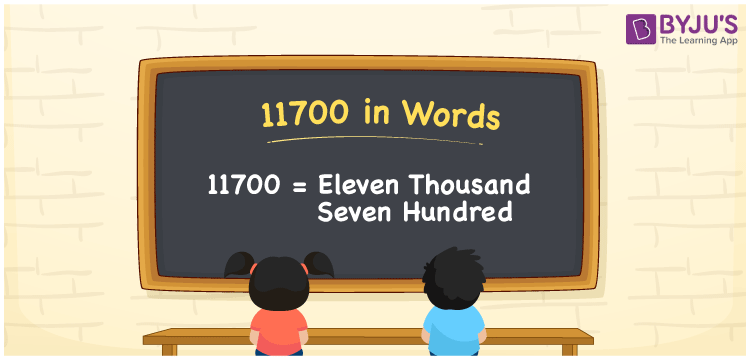# 11700 in Words

11700 in words can be written as Eleven Thousand Seven Hundred. In the article, you will understand how numbers can be written in words using the English alphabet. If you buy musical instruments for Rs. 11700, then you can say that “I bought musical instruments for Eleven Thousand Seven Hundred Rupees”. The concept of numbers in words is prepared by the experienced faculty at BYJU’S based on the understanding abilities of students. 11700 can be read as “Eleven Thousand Seven Hundred” in English.

 11700 in words Eleven Thousand Seven Hundred Eleven Thousand Seven Hundred in Numbers 11700

## 11700 in English Words## How to Write 11700 in Words?

You will learn in brief regarding the place value chart of 11700. The number 11700 has four digits and the place value of each of the digits is indicated below.

 Ten Thousands Thousands Hundreds Tens Ones 1 1 7 0 0

11700 can be written in expanded form as:

1 x Ten Thousand + 1 x Thousand + 7 × Hundred + 0 × Ten + 0 × One

= 1 x 10000 + 1 x 1000 + 7 × 100 + 0 × 10 + 0 × 1

= 10000 + 1000 + 700

= 11700

= Eleven Thousand Seven Hundred

Hence, 11700 in words is written as Eleven Thousand Seven Hundred.

11700 is a natural number that precedes 11701 and succeeds 11699.

11700 in words – Eleven Thousand Seven Hundred

Is 11700 an odd number? – No

Is 11700 an even number? – Yes

Is 11700 a perfect square number? – No

Is 11700 a perfect cube number? – No

Is 11700 a prime number? – No

Is 11700 a composite number? – Yes

## Frequently Asked Questions on 11700 in Words

### How do you write 11700 in words?

11700 can be written in words as “Eleven Thousand Seven Hundred”.

### Write Eleven Thousand Seven Hundred in numbers.

Eleven Thousand Seven Hundred in numbers can be written as 11700.

### Is 11700 an even number?

11700 is an even number as it is completely divisible by 2.